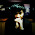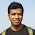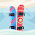## Median of Two Sorted Arrays

There are two sorted arrays A and B of size m and n respectively. Find the median of the two sorted arrays. The overall run time complexity should be O(log (m+n)).

### Analysis:

Note that the definition of the Median:
(1) If the size of the sequence N is odd: N/2+1th element is median.
(1) If the size of the sequence N is even:  average of the N/2th and N/2+1th element is median.

So in this problem, median of the two sorted arrays is the (m+n)/2+1 th element (if m+n is odd), the average of (m+n)/2 th and (m+n)/2+1 th  (if m+n is even).
E.g.,  [1,2,3],[5,6,7], the median is (3+5)/2 = 4.0.
[1,2,3,4], [1,3,5,7,9], the median is 3.

Our task becomes finding the Kth (K or K+1, K=(m+n)/2) number in two sorted arrays, in O(log(m+n)) time constraint (what's in your mind to see log? Yes, binary search).

Similar to but slight different from binary search, we still divide K into two halves each time. Two pointers are used for each array, so that we can compare which side is smaller (?A[pa]>B[pb]).
E.g., A = [1,3,5,7,9]  B = [2,4,8,10,12,14,16,18]. K=(5+8) /2= 6.

pa = K/2 = 3;
pb = K-pa = 3;
pa
A[1,3,5,7,9]
pb
B[2,4,8,10,12,14,16,18]

if (A[pa]<B[pb]), which means the elements from A to A[pa] must exist in the first Kth elements.
The next step now becomes finding the (K-pa) th (equals to K/2) element in the array A[pa:end] and B[].  This procedure can be viewed as "cutting" K/2 elements from the "smaller" array, and continue find the other K/2 th elements from the "bigger" array and the array after the cut. Note that smaller and bigger here is the comparison of the last elements.

if (A[pa]>B[pb]), the same procedure is applied but we "cut" the B array.

In this way, every time we have "cut" K/2 elements from one of the arrays, the next time is to cut (K/2) /2 elements from the new arrays, until:
(1) K=1, the smaller one from A and B is the "Kth element".
(2) One of the array meets the end. Then just return the current Kth element from the other array.

Misc. In C++, the array pointer is relative easy, (1) the array alone name represents the pointer pointed to the first element of the array, and pointer+n points to the nth element of the array. e.g.
double  arr = {1.0,2.0,3.0};
double *p = arr;        // *p=arr=1.0;
p=p+2;                     //*p=arr=3.0;

### Code(C++):

class Solution {
public:

double fms(int A[], int m, int B[], int n, int k){

if (m>n) {return fms(B,n,A,m,k);}

if (m==0) { return B[k-1];}
if (k==1) { return min(A,B);}
int pa = min(k/2,m);
int pb = k-pa;
if (A[pa-1]<=B[pb-1]) {return fms(A+pa,m-pa,B,n,k-pa);}
return fms(A,m,B+pb,n-pb,k-pb);
}

double findMedianSortedArrays(int A[], int m, int B[], int n) {
// Start typing your C/C++ solution below
// DO NOT write int main() function
int total = m + n;
if(total%2==1){
return fms(A,m,B,n,total/2+1);
}else{
return (fms(A,m,B,n,total/2)+fms(A,m,B,n,total/2+1))/2;
}

}
};


### Code(Python):

class Solution:
# @return a float
def findMedianSortedArrays(self, A, B):
if (len(A) + len(B)) % 2 == 0:
return (self.fms(A, B, (len(A) + len(B))/2) + self.fms(A, B, (len(A) + len(B))/2 + 1))/2.0
else:
return self.fms(A, B, (len(A) + len(B))/2 + 1)

def fms(self, A, B, k):
if len(A) > len(B):
return self.fms(B, A, k)
else:
if len(A) == 0:
return B[k-1]
if k == 1:
return min(A, B)
pa = min(k/2, len(A))
pb = k - pa
if A[pa-1] <= B[pb-1]:
return self.fms(A[pa::], B, k-pa)
else:
return self.fms(A, B[pb::], k-pb)



1.This comment has been removed by the author.

2.3.4.Thank you for this concise answer. I think this version is the best I can find!

5.6.Very Good Thanks

7.very concise !!

8.this algorithm is not always log(m+n). You should also choose the other half branch of A or B.
if A[pa-1] < B[pb-1]:
return float(self.fms(A[pa:], B[:pb], k - pa))
else:
return float(self.fms(A[:pa], B[pb:], k-pb))

9.This comment has been removed by the author.

10.Just a minor fix needed: When A and/or B are empty arrays k could be -1.

1.For the python code

11.Will you mind to update the CPP solution so it will be in O(min(log(n),log(m))? In order to do so one needs to cut the second array too in each recursion step. I'm struggling with with the implementation :-(

Thanks!